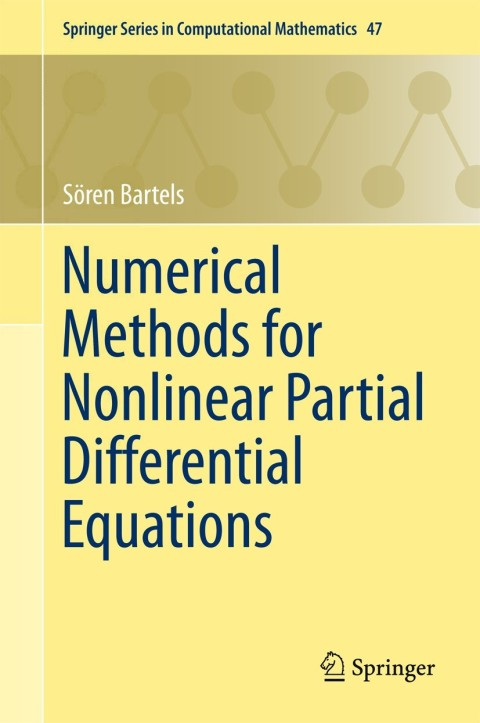# Numerical Methods for Nonlinear Partial Differential Equations

Sören Bartels   ·  ISBN 9783319137971Zookal account needed
Get it instantly
\$209.00  Save \$10.37
\$198.63
 Publisher Springer Nature Author(s) Sören Bartels Published 01192015 Related course codes
The description of many interesting phenomena in science and engineering leads to infinite-dimensional minimization or evolution problems that define nonlinear partial differential equations. While the development and analysis of numerical methods for linear partial differential equations is nearly complete, only few results are available in the case of nonlinear equations. This monograph devises numerical methods for nonlinear model problems arising in the mathematical description of phase transitions, large bending problems, image processing, and inelastic material behavior. For each of these problems the underlying mathematical model is discussed, the essential analytical properties are explained, and the proposed numerical method is rigorously analyzed. The practicality of the algorithms is illustrated by means of short implementations.